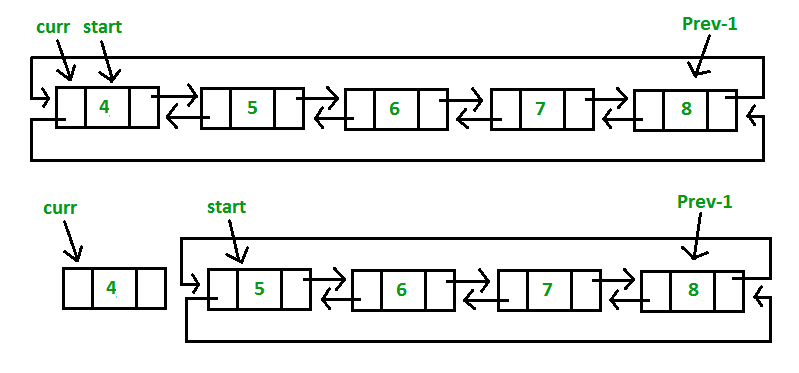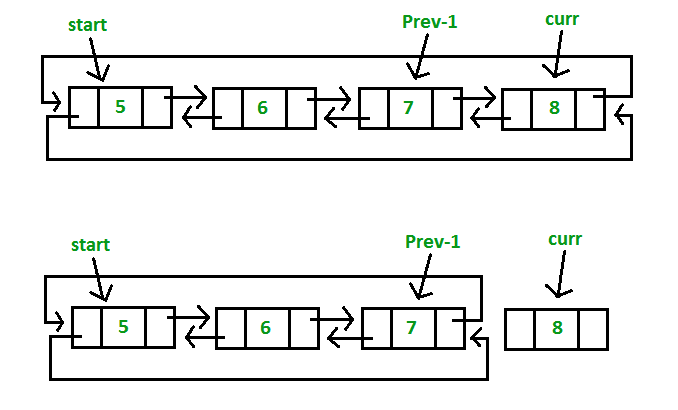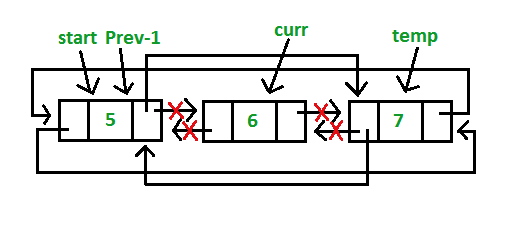Doubly Circular Linked List | Set 2 (Deletion)

• Difficulty Level : Basic
• Last Updated : 17 Aug, 2021

We have discussed doubly circular linked list introduction and its insertion.
Let us formulate the problem statement to understand the deletion process. Given a ‘key’, delete the first occurrence of this key in the circular doubly linked list.

Algorithm

Case 1: Empty List(start = NULL)

• If the list is empty, simply return it.

Case 2: The List initially contains some nodes, start points at the first node of the List

1. If the list is not empty, then we define two pointers curr and prev_1 and initialize the pointer curr points to the first node of the list and prev_1 = NULL.
2. Traverse the list using the curr pointer to find the node to be deleted and before moving from curr to the next node, every time set prev_1 = curr.
3. If the node is found, check if it is the only node in the list. If yes, set start = NULL and free the node pointing by curr.
4. If the list has more than one node, check if it is the first node of the list. The condition to check this is (curr == start). If yes, then move prev_1 to the last node(prev_1 = start -> prev). After prev_1 reaches the last node, set start = start -> next and prev_1 -> next = start and start -> prev = prev_1. Free the node pointing by curr.
5. If curr is not the first node, we check if it is the last node in the list. The condition to check this is (curr -> next == start). If yes, set prev_1 -> next = start and start -> prev = prev_1. Free the node pointing by curr.
6. If the node to be deleted is neither the first node nor the last node, declare one more pointer temp and initialize the pointer temp points to the next of curr pointer (temp = curr->next). Now set, prev_1 -> next = temp and temp ->prev = prev_1. Free the node pointing by curr.
• If the given key(Say 4) matches with the first node of the list(Step 4):• If the given key(Say 8) matches with the last node of the list(Step 5):• If the given key(Say 6) matches with the middle node of the list(Step 6):C++

 // C++ program to delete a given key from// circular doubly linked list.#include using namespace std; // Structure of a Nodestruct Node {    int data;    struct Node* next;    struct Node* prev;}; // Function to insert node in the listvoid insert(struct Node** start, int value){    // If the list is empty, create a single node    // circular and doubly list    if (*start == NULL) {        struct Node* new_node = new Node;        new_node->data = value;        new_node->next = new_node->prev = new_node;        *start = new_node;        return;    }     // If list is not empty     /* Find last node */    Node* last = (*start)->prev;     // Create Node dynamically    struct Node* new_node = new Node;    new_node->data = value;     // Start is going to be next of new_node    new_node->next = *start;     // Make new node previous of start    (*start)->prev = new_node;     // Make last previous of new node    new_node->prev = last;     // Make new node next of old last    last->next = new_node;} // Function to delete a given node from the listvoid deleteNode(struct Node** start, int key){    // If list is empty    if (*start == NULL)        return;     // Find the required node    // Declare two pointers and initialize them    struct Node *curr = *start, *prev_1 = NULL;    while (curr->data != key) {        // If node is not present in the list        if (curr->next == *start) {            printf("\nList doesn't have node with value = %d", key);            return;        }         prev_1 = curr;        curr = curr->next;    }     // Check if node is the only node in list    if (curr->next == *start && prev_1 == NULL) {        (*start) = NULL;        free(curr);        return;    }     // If list has more than one node,    // check if it is the first node    if (curr == *start) {        // Move prev_1 to last node        prev_1 = (*start)->prev;         // Move start ahead        *start = (*start)->next;         // Adjust the pointers of prev_1 and start node        prev_1->next = *start;        (*start)->prev = prev_1;        free(curr);    }     // check if it is the last node    else if (curr->next == *start) {        // Adjust the pointers of prev_1 and start node        prev_1->next = *start;        (*start)->prev = prev_1;        free(curr);    }    else {        // create new pointer, points to next of curr node        struct Node* temp = curr->next;         // Adjust the pointers of prev_1 and temp node        prev_1->next = temp;        temp->prev = prev_1;        free(curr);    }} // Function to display list elementsvoid display(struct Node* start){    struct Node* temp = start;     while (temp->next != start) {        printf("%d ", temp->data);        temp = temp->next;    }    printf("%d ", temp->data);} // Driver program to test above functionsint main(){    // Start with the empty list    struct Node* start = NULL;     // Created linked list will be 4->5->6->7->8    insert(&start, 4);    insert(&start, 5);    insert(&start, 6);    insert(&start, 7);    insert(&start, 8);     printf("List Before Deletion: ");    display(start);     // Delete the node which is not present in list    deleteNode(&start, 9);    printf("\nList After Deletion: ");    display(start);     // Delete the first node    deleteNode(&start, 4);    printf("\nList After Deleting %d: ", 4);    display(start);     // Delete the last node    deleteNode(&start, 8);    printf("\nList After Deleting %d: ", 8);    display(start);     // Delete the middle node    deleteNode(&start, 6);    printf("\nList After Deleting %d: ", 6);    display(start);     return 0;}

Java

 // Java program to delete a given key from// circular doubly linked list.class GFG {     // structure of a Node    static class Node {        int data;        Node next;        Node prev;    };     // Function to insert node in the list    static Node insert(Node start, int value)    {        // If the list is empty, create a single node        // circular and doubly list        if (start == null) {            Node new_node = new Node();            new_node.data = value;            new_node.next = new_node.prev = new_node;            start = new_node;            return start;        }         // If list is not empty         // Find last node /        Node last = (start).prev;         // Create Node dynamically        Node new_node = new Node();        new_node.data = value;         // Start is going to be next of new_node        new_node.next = start;         // Make new node previous of start        (start).prev = new_node;         // Make last previous of new node        new_node.prev = last;         // Make new node next of old last        last.next = new_node;        return start;    }     // Function to delete a given node from the list    static Node deleteNode(Node start, int key)    {        // If list is empty        if (start == null)            return null;         // Find the required node        // Declare two pointers and initialize them        Node curr = start, prev_1 = null;        while (curr.data != key) {            // If node is not present in the list            if (curr.next == start) {                System.out.printf("\nList doesn't have node with value = %d", key);                return start;            }             prev_1 = curr;            curr = curr.next;        }         // Check if node is the only node in list        if (curr.next == start && prev_1 == null) {            (start) = null;            return start;        }         // If list has more than one node,        // check if it is the first node        if (curr == start) {            // Move prev_1 to last node            prev_1 = (start).prev;             // Move start ahead            start = (start).next;             // Adjust the pointers of prev_1 and start node            prev_1.next = start;            (start).prev = prev_1;        }         // check if it is the last node        else if (curr.next == start) {            // Adjust the pointers of prev_1 and start node            prev_1.next = start;            (start).prev = prev_1;        }        else {            // create new pointer, points to next of curr node            Node temp = curr.next;             // Adjust the pointers of prev_1 and temp node            prev_1.next = temp;            temp.prev = prev_1;        }        return start;    }     // Function to display list elements    static void display(Node start)    {        Node temp = start;         while (temp.next != start) {            System.out.printf("%d ", temp.data);            temp = temp.next;        }        System.out.printf("%d ", temp.data);    }     // Driver program to test above functions    public static void main(String args[])    {        // Start with the empty list        Node start = null;         // Created linked list will be 4.5.6.7.8        start = insert(start, 4);        start = insert(start, 5);        start = insert(start, 6);        start = insert(start, 7);        start = insert(start, 8);         System.out.printf("List Before Deletion: ");        display(start);         // Delete the node which is not present in list        start = deleteNode(start, 9);        System.out.printf("\nList After Deletion: ");        display(start);         // Delete the first node        start = deleteNode(start, 4);        System.out.printf("\nList After Deleting %d: ", 4);        display(start);         // Delete the last node        start = deleteNode(start, 8);        System.out.printf("\nList After Deleting %d: ", 8);        display(start);         // Delete the middle node        start = deleteNode(start, 6);        System.out.printf("\nList After Deleting %d: ", 6);        display(start);    }} // This code is contributed by Arnab Kundu

Python3

 # Python3 program to delete a given key from# circular doubly linked list. # structure of a node of linked listclass Node:    def __init__(self, data):        self.data = data        self.next = None        self.prev = None def insert( start, value):         # If the list is empty, create a single node    # circular and doubly list    if (start == None):        new_node = Node(0)        new_node.data = value        new_node.next = new_node.prev = new_node        start = new_node        return start             # If list is not empty     # Find last node /    last = (start).prev     # Create Node dynamically    new_node = Node(0)    new_node.data = value     # Start is going to be next of new_node    new_node.next = start     # Make new node previous of start    (start).prev = new_node     # Make last previous of new node    new_node.prev = last     # Make new node next of old last    last.next = new_node    return start     # Function to delete a given node# from the listdef deleteNode(start, key):         # If list is empty    if (start == None):        return None     # Find the required node    # Declare two pointers and initialize them    curr = start    prev_1 = None    while (curr.data != key) :                 # If node is not present in the list        if (curr.next == start) :            print ("List doesn't have node",                       "with value = ", key)            return start                     prev_1 = curr        curr = curr.next             # Check if node is the only node in list    if (curr.next == start and prev_1 == None) :        (start) = None        return start             # If list has more than one node,    # check if it is the first node    if (curr == start) :                 # Move prev_1 to last node        prev_1 = (start).prev         # Move start ahead        start = (start).next         # Adjust the pointers of prev_1        # and start node        prev_1.next = start        (start).prev = prev_1             # check if it is the last node    elif (curr.next == start) :                 # Adjust the pointers of prev_1        # and start node        prev_1.next = start        (start).prev = prev_1             else :                 # create new pointer,        # points to next of curr node        temp = curr.next         # Adjust the pointers of prev_1        # and temp node        prev_1.next = temp        temp.prev = prev_1             return start     # Function to display list elementsdef display(start):         temp = start     while (temp.next != start) :        print (temp.data, end = " ")        temp = temp.next             print (temp.data)     # Driver Codeif __name__=='__main__':         # Start with the empty list    start = None     # Created linked list will be 4.5.6.7.8    start = insert(start, 4)    start = insert(start, 5)    start = insert(start, 6)    start = insert(start, 7)    start = insert(start, 8)     print ("List Before Deletion: ")    display(start)     # Delete the node which is not present in list    start = deleteNode(start, 9)    print ("List After Deletion: ")    display(start)     # Delete the first node    start = deleteNode(start, 4)    print ("List After Deleting", 4)    display(start)     # Delete the last node    start = deleteNode(start, 8)    print ("List After Deleting ", 8)    display(start)     # Delete the middle node    start = deleteNode(start, 6)    print ("List After Deleting ", 6)    display(start)     # This code is contributed by Arnab Kundu

C#

 // C# program to delete a given key from// circular doubly linked list.using System; class GFG {     // structure of a Node    public class Node {        public int data;        public Node next;        public Node prev;    };     // Function to insert node in the list    static Node insert(Node start, int value)    {        // If the list is empty, create a single node        // circular and doubly list        Node new_node = new Node();        if (start == null) {             new_node.data = value;            new_node.next = new_node.prev = new_node;            start = new_node;            return start;        }         // If list is not empty         // Find last node /        Node last = (start).prev;         // Create Node dynamically        new_node = new Node();        new_node.data = value;         // Start is going to be next of new_node        new_node.next = start;         // Make new node previous of start        (start).prev = new_node;         // Make last previous of new node        new_node.prev = last;         // Make new node next of old last        last.next = new_node;        return start;    }     // Function to delete a given node from the list    static Node deleteNode(Node start, int key)    {        // If list is empty        if (start == null)            return null;         // Find the required node        // Declare two pointers and initialize them        Node curr = start, prev_1 = null;        while (curr.data != key) {            // If node is not present in the list            if (curr.next == start) {                Console.Write("\nList doesn't have node with value = {0}", key);                return start;            }             prev_1 = curr;            curr = curr.next;        }         // Check if node is the only node in list        if (curr.next == start && prev_1 == null) {            (start) = null;            return start;        }         // If list has more than one node,        // check if it is the first node        if (curr == start) {            // Move prev_1 to last node            prev_1 = (start).prev;             // Move start ahead            start = (start).next;             // Adjust the pointers of prev_1 and start node            prev_1.next = start;            (start).prev = prev_1;        }         // check if it is the last node        else if (curr.next == start) {            // Adjust the pointers of prev_1 and start node            prev_1.next = start;            (start).prev = prev_1;        }        else {            // create new pointer, points to next of curr node            Node temp = curr.next;             // Adjust the pointers of prev_1 and temp node            prev_1.next = temp;            temp.prev = prev_1;        }        return start;    }     // Function to display list elements    static void display(Node start)    {        Node temp = start;         while (temp.next != start) {            Console.Write("{0} ", temp.data);            temp = temp.next;        }        Console.Write("{0} ", temp.data);    }     // Driver code    public static void Main(String[] args)    {        // Start with the empty list        Node start = null;         // Created linked list will be 4.5.6.7.8        start = insert(start, 4);        start = insert(start, 5);        start = insert(start, 6);        start = insert(start, 7);        start = insert(start, 8);         Console.Write("List Before Deletion: ");        display(start);         // Delete the node which is not present in list        start = deleteNode(start, 9);        Console.Write("\nList After Deletion: ");        display(start);         // Delete the first node        start = deleteNode(start, 4);        Console.Write("\nList After Deleting {0}: ", 4);        display(start);         // Delete the last node        start = deleteNode(start, 8);        Console.Write("\nList After Deleting {0}: ", 8);        display(start);         // Delete the middle node        start = deleteNode(start, 6);        Console.Write("\nList After Deleting {0}: ", 6);        display(start);    }} // This code has been contributed by 29AjayKumar

Javascript



Output:

List Before Deletion: 4 5 6 7 8
List doesn't have node with value = 9
List After Deletion: 4 5 6 7 8
List After Deleting 4: 5 6 7 8
List After Deleting 8: 5 6 7
List After Deleting 6: 5 7

This article is contributed by Akash Gupta. If you like GeeksforGeeks and would like to contribute, you can also write an article using write.geeksforgeeks.org or mail your article to review-team@geeksforgeeks.org. See your article appearing on the GeeksforGeeks main page and help other Geeks.